# Abstract

We compute the Casimir energy for a massive scalar field constrained between two parallel planes (Dirichlet boundary conditions) in order to investigate its non-relativistic limit. Instead of employing the usual relativistic dispersion relation omega(p) = <img SRC="http:/img/fbpe/bjp/v31n1/08eq01.gif">, we use the non-relativistic one, omega(p) = p²/2m. It turns out that the Casimir energy is zero. We include the relativistic corrections perturbatively and show that at all orders the Casimir energy remains zero, since each term in the power series in 1/c² is proportional to the Riemann zeta function of a negative even integer. This puzzling result shows that, at least for the free massive scalar field, the Casimir effect is non-perturbative in the relativistic sense.

On the non-relativistic Casimir effect

M.V. Cougo-Pinto* * e-mail: marcus@if.ufrj.br  e-mail: farina@if.ufrj.br  e-mail: mendes@if.ufrj.br § e-mail: tort@if.ufrj.br , C. Farina * e-mail: marcus@if.ufrj.br  e-mail: farina@if.ufrj.br  e-mail: mendes@if.ufrj.br § e-mail: tort@if.ufrj.br , J.F.M. Mendes * e-mail: marcus@if.ufrj.br  e-mail: farina@if.ufrj.br  e-mail: mendes@if.ufrj.br § e-mail: tort@if.ufrj.br , A.C. Tort§ * e-mail: marcus@if.ufrj.br  e-mail: farina@if.ufrj.br  e-mail: mendes@if.ufrj.br § e-mail: tort@if.ufrj.br

Instituto de Física, Universidade Federal do Rio de Janeiro

CP 68528, Rio de Janeiro, RJ 21945-970, Brazil

Received on 7 June, 2000. Revised version received on 29 July, 2000

We compute the Casimir energy for a massive scalar field constrained between two parallel planes (Dirichlet boundary conditions) in order to investigate its non-relativistic limit. Instead of employing the usual relativistic dispersion relation w(

p

) =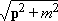, we use the non-relativistic one, w(

p

) =

p2

/

2

m

. It turns out that the Casimir energy is zero. We include the relativistic corrections perturbatively and show that at all orders the Casimir energy remains zero, since each term in the power series in 1/

c

2

is proportional to the Riemann zeta function of a negative even integer. This puzzling result shows that, at least for the free massive scalar field, the Casimir effect is non-perturbative in the relativistic sense.

In 1948 Casimir  calculated the shift in the zero point energy of the quantum electromagnetic field caused by the presence of two perfectly conducting plates, parallel to each other and kept at a small distance apart from each other so that edge effects can be neglected. Assuming that the vacuum energy vanishes in the absence of the material plates and denoting bythe vacuum energy shift caused by the plates, the result obtained by Casimir is given by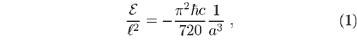whereis the linear dimension of the plates, supposed to be identical and to take the form of perfect squares; a is the separation between the plates and the condition a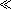is assumed. The dependence of the energy shifton the separation between the plates leads to a force per unit area between the plates which by virtue of equation (1) is given byThe Casimir force given by (2) was measured for the first time by Sparnaay  who cautiously stated that the experimental results were not incompatible with the theory. Recently, however, new experiments performed independently by Lamoreaux  and Mohideen and Roy [4, 5] greatly increased our confidence in the reality of the Casimir effect. From a theoretical viewpoint, any influence on the quantum vacuum fluctuations due to a non-trivial topology, that is, one different from the familar3 topology, goes under the common name of Casimir effect . In the original Casimir effect the introduction of the plates turn the trivial3 topology of the free space into a2 × [0, a] topology. With this broader significance the Casimir effect plays a major role in quantum field theories and has applications, for instance, in cosmology and gravitation.

The Casimir effect has a special place in modern physics due to its experimental actuality and due to the fact that it deals with one of the most fundamental concepts of quantum field theory: the quantum vacuum. On the other hand, the significance and interpretation of this effect are not controversy free. In particular, there is the question of whether or not the effect, which is a genuine quantum effect, shows up only in the relativistic context. In fact, the original (electromagnetic) Casimir effect is intrinsically relativistic, since the photon is a massless particle. Consequently, there is no meaning in taking the limit c ® ¥ in (1). Moreover, the Casimir effect is usually calculated and interpreted in a relativistic way, i. e., as a consequence of the modifications in the fluctuations of virtual particle-antiparticle pairs due to the imposition of external conditions. In the case of a non-relativistic quantum field there are no antiparticles, therefore the ordering problem which gives rise to the vaccum energy is absent. From this fundamental point of view there is no Casimir energy for such a field. However, we can consider a meaningful non-relativistic limit of some quantum field and ask what happens to the Casimir energy when we approach such a limit. If continuity holds we would expect a diminishing Casimir energy. We can consider a relativistic massive quantum field, for which a Casimir energy does exist, and take the limit of large mass which leads to the usual non-relativistic approximation. Hopefully a zero limit for the Casimir energy will be obtained in this case. If this is the case the next step is to look for the relativistic corrections to this zero energy, in order to understand how the zero limit is approached. A naïve expectation would be a small Casimir energy for a slightly relativistic theory. We treat those matters below, where we obtain a zero non-relativistic limit for the Casimir energy, in agreement with the above remark, but also an unexpected and rather subtle result for the order by order relativistic contributions to the Casimir energy. We shall show that the relativistic corrections to any finite order (in powers of p2/m2c2) vanish, since each of them separately will be proportional to a Riemann zeta function of a negative even integer.

Let us consider for simplicity a neutral scalar massive field. Let us also consider the vacuum of this field distorted by two parallel surfaces. We impose Dirichlet boundary conditions on the field at the location of these surfaces, exactly as in the original Casimir effect. Our first calculation will be with the non-relativistic dispersion relation, that is,w(p) = p2/2m. The imposition of Dirichlet boundary conditions on the field results in the discretization of the component of the linear momentum perpendicular to the surfaces, that is p^ = np/a with n Î lN ={1, 2, 3, ...}. The component parallel to the surfaces is free to assume any value in2. The Casimir energy of this field can be easily calculated by adding the energies associated with the allowed modes of the field and making use of a regularization recipe. The regularization recipe is a matter of convenience and taste, but the physical result must be the same for any recipe. The ensuing calculations are well-known in the literature [6, 7, 8] and so are some results of classical analysis  that we take advantage of. The regularized expression for the energy iswhere we included negative powers of p2/2m in order to obtain a regularized expression for the zero point energy. The factor L2s was inserted to ensure dimensional coherence. The parameter s is large enough to ensure that the above expression is well defined. After an analytical extension of(a, s) to the whole complex plane of s (or at least to a domain containing the origin) we obtain the physical result taking the limit s ® 0. Using a well known integral representation of the Euler Beta function  we obtainwhere we have identified the Riemann zeta function. In fact, z must be thought here as the analytical extension of the Riemann zeta function to the whole complex plane. Since its unique pole occurs when its argument is equal to one, which means in the previous equation s = 5/2, there will be no problem in taking the limit s ® 0 in this equation. The non-relativistic Casimir energy is then given by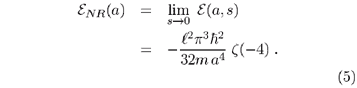Therefore, this non-relativistic Casimir energy is zero, for the Riemann zeta function is zero for any even negative integer.

Let us now consider the first relativistic corrections, that is, let us include in the calculation of the zero point energy those terms stemming from the series development of the relativistic dispersion relation in powers of p2/m2c2 up to order N. The first term of this series, after subtracting the rest mass term, is precisely the contribution just computed and given by equation (5). The relativistic corrections are easily obtained by expanding the relativistic dispersion relation:The improved Casimir energy containing the first N relativistic corrections is then given bywithwhere bj are the binomial coefficients of the expansion and the regulator parameter s, after an analytical extension of the previous expression and further elimination of spurious terms, must again assume a value equal to 0. It is not difficult to show that when s ® 0 then Tj(a, s) will be proportional to z(2js - 2j - 2) and we can conclude that Tj(a, s)=z(-2j - 2)=0 for j=1, 2... N and arbitrary N. This result shows that the Casimir effect for a massive scalar field, which is a quantum effect, is also intrinsically a relativistic one. In other words, retarded forces are intrinsically relativistic in the sense that any finite series expansion in powers of 1/c will never generate perturbatively the Casimir effect.

A possible interpretation of this rather unexpected result is the following: let us consider the full relativistic Casimir effect for a massive scalar field with the same boundary conditions as before. The corresponding Casimir energy is given by (see also a very nice discussion of the 1+1 dimensional case in )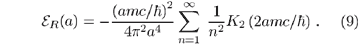Since the non-relativistic limit can be achieved by taking c ® ¥, or equivalently, 1/c ® 0, let us write an expression that approximates the previous formula for large values of amc/:where we have written conveniently the exponent as a function of the variable 1/c. Notice that: (i) in the limit c ® ¥ the Casimir energy vanishes, in agreement with our previous calculation; (ii) the expression (10) is not analytic in the variable 1/c at 1/c=0, so that there is no way of obtaining this expression through a power series in 1/c. This result is analogous to what happens in the pair creation phenomenon by a uniform electric field E, where the expression for the pair creation probability rate G, namely [11, 12],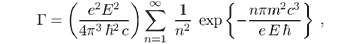is not analytic in the coupling constant e at e = 0, so that the result can not be obtained by a perturbative approach [11, 12]. Pair creation is not a perturbative phenomenon. Similarly, we can say that the Casimir effect is also a non-perturbative effect in the relativistic sense. As a final remark, we must say that if we use another regularization prescription, as for example the cutoff method, the same result will be obtained.

Acknowledgments

The authors thank M.B. Silva Neto for enlightning discussions. M. V. Cougo-Pinto and C. Farina acknowledge partial financial support of the CNPq (The National Reaserch Council of Brazil).

References

 H. B. G. Casimir, Proc. K. Ned. Akad. Wet. 51, 793 (1948).

 M. J. Sparnaay, Physica XXXIV, 751 (1958).

 S. K. Lamoreaux, Phys. Rev Lett. 78, 5 (1997).

 U. Mohideen and A. Roy, Phys. Rev. Lett. 81, 4549 (1998).

 A. Roy and U. Mohideen, Phys. Rev. Lett. 82, 4380 (1999).

 V. M. Mostepanenko and N. N. Trunov, The Casimir effect and its Applications (Oxford: Clarendon, 1997); V. M. Mostepanenko and N. N. Trunov Sov. Phys. Usp. 31 965 (1988).

 G. Plunien, B. Müller and W. Greiner, Phys. Rep. 134, 89 (1986).

 P. W. Milonni, The Quantum Vacuum: an Introduction to Quantum Electrodynamics, Academic Press (1992).

 I. S. Gradshtejn and I. M. Ryzhik Tables of Integrals, Series and Products (New York: Academic Press, 1965).

 P. Hays, Ann. Phys. (N.Y.) 121, 32 (1979).

 J. Schwinger, Phys. Rev. 82, 664 (1951).

 W.Dittrich and M. Reuter, Effective Lagrangians in Quantum Electrodynamics (Berlin: Springer-Verlag, 1985) 220.

•  H. B. G. Casimir, Proc. K. Ned. Akad. Wet. 51, 793 (1948).
•  M. J. Sparnaay, Physica XXXIV, 751 (1958).
•  S. K. Lamoreaux, Phys. Rev Lett. 78, 5 (1997).
•  U. Mohideen and A. Roy, Phys. Rev. Lett. 81, 4549 (1998).
•  A. Roy and U. Mohideen, Phys. Rev. Lett. 82, 4380 (1999).
•  V. M. Mostepanenko and N. N. Trunov, The Casimir effect and its Applications (Oxford: Clarendon, 1997);
• V. M. Mostepanenko and N. N. Trunov Sov. Phys. Usp. 31 965 (1988).
•  G. Plunien, B. Müller and W. Greiner, Phys. Rep. 134, 89 (1986).
•  P. W. Milonni, The Quantum Vacuum: an Introduction to Quantum Electrodynamics, Academic Press (1992).
•  I. S. Gradshtejn and I. M. Ryzhik Tables of Integrals, Series and Products (New York: Academic Press, 1965).
•  P. Hays, Ann. Phys. (N.Y.) 121, 32 (1979).
•  J. Schwinger, Phys. Rev. 82, 664 (1951).
•  W.Dittrich and M. Reuter, Effective Lagrangians in Quantum Electrodynamics (Berlin: Springer-Verlag, 1985) 220
• *
e-mail:

e-mail:

e-mail:
§
e-mail:

# Publication Dates

• Publication in this collection
18 Feb 2002
• Date of issue
Mar 2001

# History

• Received
07 June 2000
• Reviewed
29 July 2000
Sociedade Brasileira de Física Caixa Postal 66328, 05315-970 São Paulo SP - Brazil, Tel.: +55 11 3091-6922, Fax: (55 11) 3816-2063 - São Paulo - SP - Brazil
E-mail: sbfisica@sbfisica.org.br